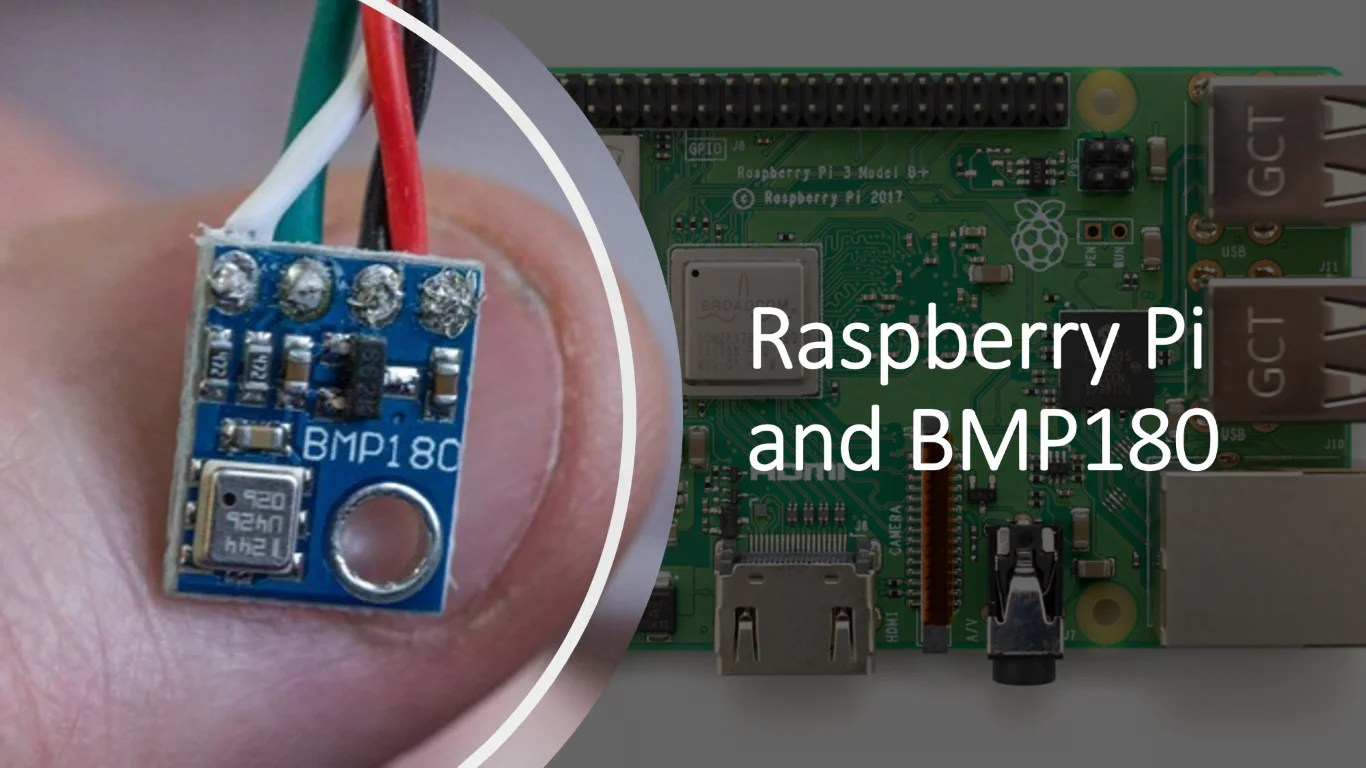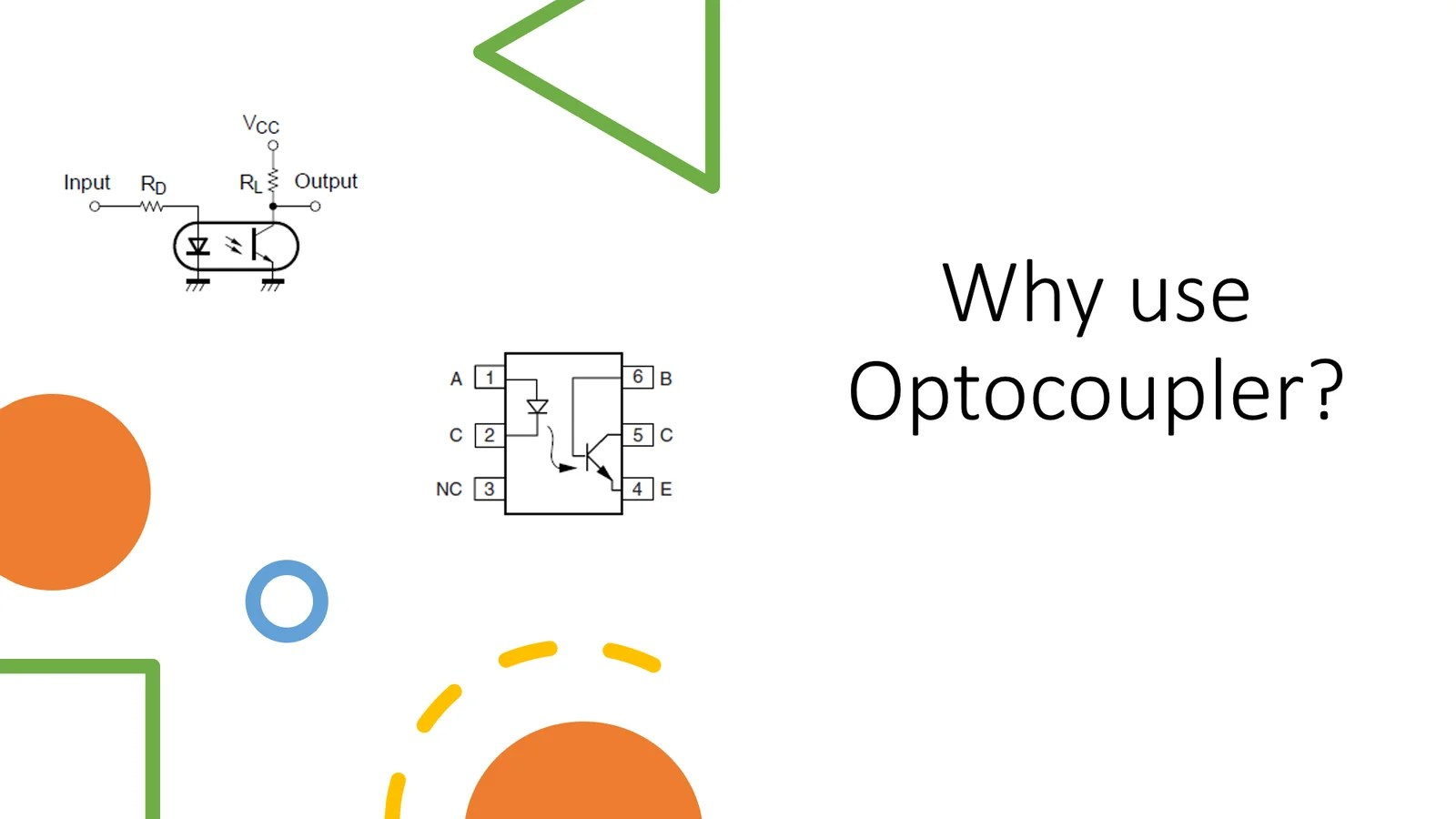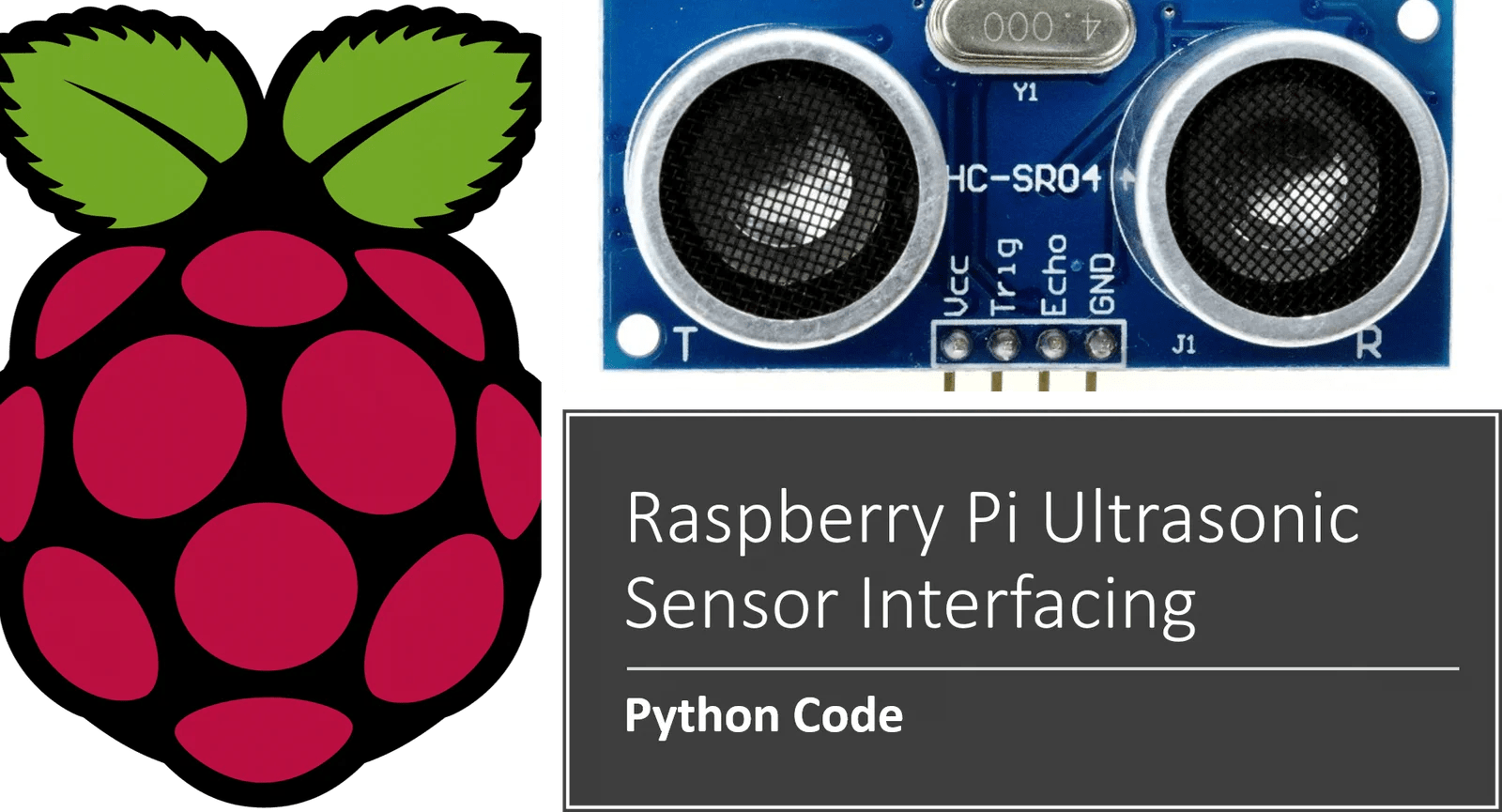Select Page

## Protected: Auto Draft

To view this protected post, enter the password below:## Raspberry Pi with BMP180 Sensor Interfacing

BMP180 is a nice tiny sensor which can give the values of

• Atmospheric Pressure
• Temperature
• Altitude

In this video, we’ll see how we can interface Raspberry pi with BMP180 and how to write python code for the same.

In order to follow this video you’ll need

• Raspberry Pi 2/3/4 with Raspbian OS Installed on it
• BMP180 Sensor
• Female to Female Wire connectors

For setting up the raspberry pi and start using it, you can follow this post.

## Python Program to interface raspberry pi with BMP180.

The video shows about 2 different programs one is the library package and other is testing code. Make sure while testing out, both the programs stay in the same folder.

Here are the 2 programs

### Python Program for BMP180 Sensor Part 1

```# make sure to install python-smbus using below command
# sudo apt-get install python-smbus
import smbus
import time
from ctypes import c_short

DEVICE = 0x77 # Default device I2C address

#bus = smbus.SMBus(0)  # Rev 1 Pi uses 0
bus = smbus.SMBus(1) # Rev 2 Pi uses 1

def convertToString(data):
# Simple function to convert binary data into
# a string
return str((data + (256 * data)) / 1.2)

def getShort(data, index):
# return two bytes from data as a signed 16-bit value
return c_short((data[index] << 8) + data[index + 1]).value

def getUshort(data, index):
# return two bytes from data as an unsigned 16-bit value
return (data[index] << 8) + data[index + 1]

REG_ID     = 0xD0
return (chip_id, chip_version)

REG_CALIB  = 0xAA
REG_MEAS   = 0xF4
REG_MSB    = 0xF6
REG_LSB    = 0xF7
CRV_TEMP   = 0x2E
CRV_PRES   = 0x34
# Oversample setting
OVERSAMPLE = 3    # 0 - 3

# Read calibration data from EEPROM

# Convert byte data to word values
AC1 = getShort(cal, 0)
AC2 = getShort(cal, 2)
AC3 = getShort(cal, 4)
AC4 = getUshort(cal, 6)
AC5 = getUshort(cal, 8)
AC6 = getUshort(cal, 10)
B1  = getShort(cal, 12)
B2  = getShort(cal, 14)
MB  = getShort(cal, 16)
MC  = getShort(cal, 18)
MD  = getShort(cal, 20)

time.sleep(0.005)
UT = (msb << 8) + lsb

bus.write_byte_data(addr, REG_MEAS, CRV_PRES + (OVERSAMPLE << 6))
time.sleep(0.04)
UP = ((msb << 16) + (lsb << 8) + xsb) >> (8 - OVERSAMPLE)

# Refine temperature
X1 = ((UT - AC6) * AC5) >> 15
X2 = (MC << 11) / (X1 + MD)
B5 = X1 + X2
temperature = int(B5 + 8) >> 4
temperature = temperature / 10.0

# Refine pressure
B6  = B5 - 4000
B62 = int(B6 * B6) >> 12
X1  = (B2 * B62) >> 11
X2  = int(AC2 * B6) >> 11
X3  = X1 + X2
B3  = (((AC1 * 4 + X3) << OVERSAMPLE) + 2) >> 2

X1 = int(AC3 * B6) >> 13
X2 = (B1 * B62) >> 16
X3 = ((X1 + X2) + 2) >> 2
B4 = (AC4 * (X3 + 32768)) >> 15
B7 = (UP - B3) * (50000 >> OVERSAMPLE)

P = (B7 * 2) / B4

X1 = (int(P) >> 8) * (int(P) >> 8)
X1 = (X1 * 3038) >> 16
X2 = int(-7357 * P) >> 16
pressure = int(P + ((X1 + X2 + 3791) >> 4))
#pressure = float(pressure / 100.0)

altitude = 44330.0 * (1.0 - pow(pressure / 101325.0, (1.0/5.255)))
altitude = round(altitude,2)

return (temperature,pressure,altitude)
```

### Python Program for BMP180 Sensor Part 2

```import bmpsensor
import time
while True:
print("Temperature is ",temp)  # degC
print("Pressure is ",pressure) # Pressure in Pa
print("Altitude is ",altitude) # Altitude in meters
print("\n")
time.sleep(2)```

If you face any issues with the code or working or explanation, feel free to comment about it!!## Why we use Optocoupler in relay switching?

Optocoupler, a tiny IC which is basically an LED triggered transistor can be live saving in many project situation. This is a short story about one such incident in project development and am sure it’ll help you if you face such issues in your microcontroller projects

Last week we were working on an Industrial project. It wasn’t something that I had designed but had been called upon for troubleshooting the issue which was coming in. The project was simple, it had below mentioned interfaces

1. Ultrasonic Sensor
2. Microcontroller(atmega328p)
3. LCD
4. Relay x 2
5. DC Motor x 2

Primarily, the job was to turn on and off those 2 dc motors based on some sequences of distance sensed by the ultrasonic distance sensor. However these 2 DC motor were actually water pumps, and were operating on 24 volts

Now you must have known it till now that any Motor is an Inductive load and the microcontrollers are too much scared of inductive loads 😄 😄 😄

Because when they turn On, the supply Line shatters a little bit and hence the microcontroller either hangs or gets a reset.

So what was happening in that project was, whenever we turn on a Motor in program sequence, the entire system hangs. Boom, nothing else. It simply stops executing the code, the LCD displays some garbage characters.

Now there are many techniques with which these interferences may be reduced, primarily by using the Snubber Circuit.

Below is something which was present there at the time of doing this experimentNow as you can see, there is fly back diode present, there is the RC Snubber present, additionally such snubber was also connected across the com and NO of relay. But still it didn’t helped

whenever the relay was turned On by controller signal, the controller Hang.

Now there are several reasons for such thing to happen, but primarily, the reason is NOISE. And if you can’t seem to solve such thing, then its better and much better if at the time of design itself, you incorporate optocoupler.

What is optocoupler? If you ask me, its a life saver 😎😎

Its basically a replacement to transistorized switch but this time, the switching happens just by emitting light using the atmega signal and hence, the noise induced in controller side is at minimum. You can also NOT REQUIRED TO MAKE COMMON GROUND when using optocoupler. Means the 24v ground and +5v ground need not to be made common.

Finally below is the arrangement which solved all issues with the systemas you can see, the optocoupler provides complete electrical isolation between 24v side and +5v side hence this arrangement worked perfectly for the system

The optocoupler used was MCT2E, however you can use any optocoupler you may have like PC817

If there is a base Pin to your optocoupler, simple keep it open or Not connected to anything. Its just there to give a manual trigger instead of the light trigger that comes off from LED and can keep it open while using the LED inside optocoupler.

Whether your project is based on Arduino or Atmega or PIC or STM32 or Raspberry Pi, this tip will help in every situation where ever you need to trigger relays in your system, particularly when what you’re switching is a high current device

So keep this thing in mind next time when you’re debugging your relay based microcontroller project.## How to interface raspberry pi with Ultrasonic Sensor

This is a video tutorial about how to interface raspberry Pi with ultrasonic sensor. Ultrasonic sensor is used for distance measurement and gives fairly accurate readings as its using ultrasonic sound to find the distance. This is a detailed tutorial including all the explanation about interfacing of sensor, its working, and the algorithm of python program.

The python program is also explained in detail in the video.

To interface raspberry pi with ultrasonic sensor and create a distance meter, you’ll need

• Raspberry Pi any version
• Ultrasonic sensor HC-SR04
• 1k Resistor
• 1.5k Resistor
• Connecting Wires

## Circuit Diagram of Ultrasonic sensor with Raspberry Pi

The interfacing diagram is as shown below

## Python Code for Ultrasonic Sensor Interfacing with Raspberry Pi

```import RPi.GPIO as GPIO
import time
TRIG=21
ECHO=20
GPIO.setmode(GPIO.BCM)
while True:
print"distance measurement in progress"
GPIO.setup(TRIG,GPIO.OUT)
GPIO.setup(ECHO,GPIO.IN)
GPIO.output(TRIG,False)
print"waiting for sensor to settle"
time.sleep(0.2)
GPIO.output(TRIG,True)
time.sleep(0.00001)
GPIO.output(TRIG,False)
while GPIO.input(ECHO)==0:
pulse_start=time.time()
while GPIO.input(ECHO)==1:
pulse_end=time.time()
pulse_duration=pulse_end-pulse_start
distance=pulse_duration*17150
distance=round(distance,2)
print"distance:",distance,"cm"
time.sleep(2)
```

Above code is created in Python 3, however if you’re using python 2 the code will still work for you.

## Complete Video tutorial

This is the detailed tutorial of Ultrasonic Sensor Interfacing with Raspberry Pi

Keep experimenting!!!